# Galerkin method

(Redirected from Bubnov-Galerkin method)

method of moments

A method for finding the approximate solution of an operator equation in the form of a linear combination of the elements of a given linearly independent system.

Letbe a non-linear operator, with domain of definition in a Banach spaceand range of values in a Banach space. To solve the equation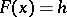(1)

by the Galerkin method one chooses a linearly independent systemof elements from(a coordinate system) and a linearly independent system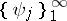of functionals from the space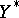dual to(a projection system). An approximate solutionof equation (1) is sought for in the form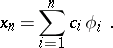(2)

The numerical coefficients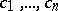are found from the system of equations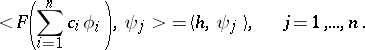(3)

When the problem is posed in this general manner, (3) need not have any solutions. If (3) has a unique solution, for eachthe approximate solution (2) need not converge, not even weakly, to the exact solution of equation (1) as. Nevertheless, Galerkin's method is a powerful tool not only for finding approximate solutions, but also for proving existence theorems of solutions of linear and non-linear equations, especially so in problems involving partial differential equations.

In many cases the problem of determining the coefficients of (2) from the system (3) is equivalent to that of finding the minimum of some functional, and Galerkin's method becomes a variational (energy) method. The most important such method is the Ritz method. In certain cases it may be expedient to use topological methods in the investigation of (3).

Ifandare Hilbert spaces, Galerkin's method is sometimes called the Petrov–Galerkin method. If, in addition, the coordinate and the projection systems are identical (and), one usually speaks of the Bubnov–Galerkin method. If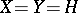is a Hilbert space, and also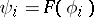, this special case is known as the method of least squares (cf. Least squares, method of).

In the linear case, when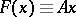, witha linear, generally unbounded, operator with domainand range, while the coordinate system has been selected in, equation (1) assumes the form(4)

Here, (3) is a system oflinear equations inunknowns:(5)

If, under the conditions of the method of least squares, there exists a bounded inverse operator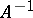on, ifand if the systemis complete in, then the approximate solution of (2) converges asto the exact solution of equation (4). If under the conditions of the Petrov–Galerkin method the operatoris symmetric and positive definite,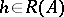and if the system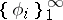is complete in the Hilbert space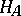(the completion of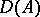in the metric generated by the scalar product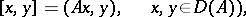then the approximate solution of (2) converges to the exact solution of (4) both inand in.

Ifis a positive-definite self-adjoint operator onand ifis a complete orthonormal system of its eigen elements, then the Bubnov–Galerkin method and the method of least squares coincide with the Fourier method.

Galerkin's method is also employed in the approximate solution of eigen value and eigen element problems.

Galerkin's method has found widespread use after the studies of B.G. Galerkin ; it was formerly used by I.G. Bubnov in solving specific problems in elasticity theory. There is a general approach to approximate methods, which includes projection methods, finite-difference methods (cf. Projection methods; Difference methods) and other approximate methods which are generalizations of Galerkin's method.